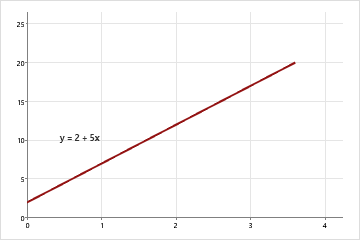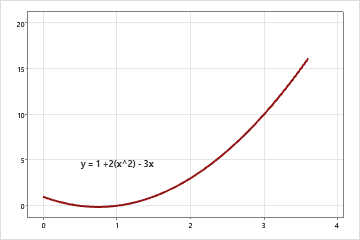# Regression coefficients

## What is a coefficient?

Coefficients are the numbers by which the variables in an equation are multiplied. For example, in the equation y = -3.6 + 5.0X1 - 1.8X2, the variables X1 and X2 are multiplied by 5.0 and -1.8, respectively, so the coefficients are 5.0 and -1.8.

The size and sign of a coefficient in an equation affect its graph. In a simple linear equation (contains only one x variable), the coefficient is the slope of the line.The coefficient (and slope) is positive 5.The coefficients are 2 and -3.

When calculating a regression equation to model data, Minitab estimates the coefficients for each predictor variable based on your sample and displays these estimates in a coefficients table. For example, the following coefficients table is shown in the output for a regression equation:

Regression Equation Heat Flux = 325.4 + 2.55 East + 3.80 South - 22.95 North + 0.0675 Insolation + 2.42 Time of Day

This equation predicts the heat flux in a home based on the position of its focal points, the insolation, and the time of day. Minitab displays the coefficient values for the equation in the second column:

Coefficients Term Coef SE Coef T-Value P-Value VIF Constant 325.4 96.1 3.39 0.003 East 2.55 1.25 2.04 0.053 1.36 South 3.80 1.46 2.60 0.016 3.18 North -22.95 2.70 -8.49 0.000 2.61 Insolation 0.0675 0.0290 2.33 0.029 2.32 Time of Day 2.42 1.81 1.34 0.194 5.37

Each coefficient estimates the change in the mean response per unit increase in X when all other predictors are held constant. For example, in the regression equation, if the North variable increases by 1 and the other variables remain the same, heat flux decreases by about 22.95 on average.

If the p-value of a coefficient is less than the chosen significance level, such as 0.05, the relationship between the predictor and the response is statistically significant. Minitab also includes a value for the constant in the equation in the Coef column.

###### Note

The term coefficient can also be used to denote a calculated numerical value used as an index, such as a coefficient of correlation, a coefficient of determination, or Kendall's coefficient.

## Calculate confidence intervals for regression coefficients

Use the confidence interval to assess the reliability of the estimate of the coefficient. The wider the confidence interval, the less precise the estimate is.

1. Choose Stat > Regression > Regression > Fit Regression Model.
2. Complete the dialog box.
3. Click Results.
4. From Display of results, choose Simple tables. Then click OK in each dialog box.
###### Note

By default, Minitab calculates 95% two-sided confidence intervals. To change the default settings, choose Stat > Regression > Regression > Fit Regression Model, then click Options. Then enter the confidence level and type of interval.

By using this site you agree to the use of cookies for analytics and personalized content.  Read our policy